# Curve Volume

Curve volumes are volumes that can be attached to NURBS curve objects. They define a tubular volume around that curve.

View-port rendering and editing is handled by a VolumeLocatorNode.

## Key Points

A curve’s volume is defined by a series of key points. Each key point defines the volume radius at that point and the volume is linearly interpolated between key points to create a continuous volume along the curve.

By adjusting the radii at these key points, it is possible to create quite intricate and detailed volume shapes.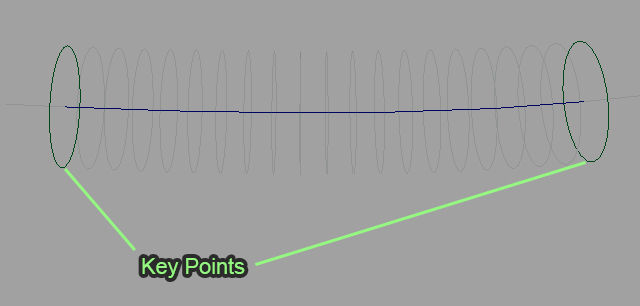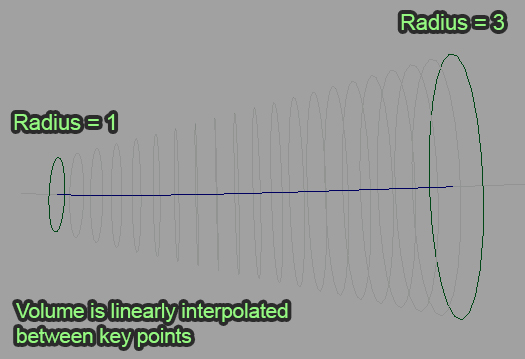## Resolution

The number of key points in a curve volume is determined by its Volume Resolution attribute. The minimum resolution which can be chosen is 2 (one key point at each end of the curve). Increasing the resolution of the curve volume will increase the number of key points along its length.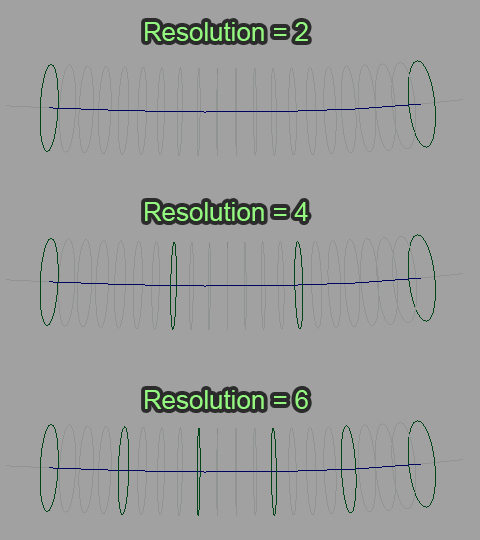Key points are spaced equally along the curve length and therefore resizing a curve will alter the positions of key points in world space as seen below: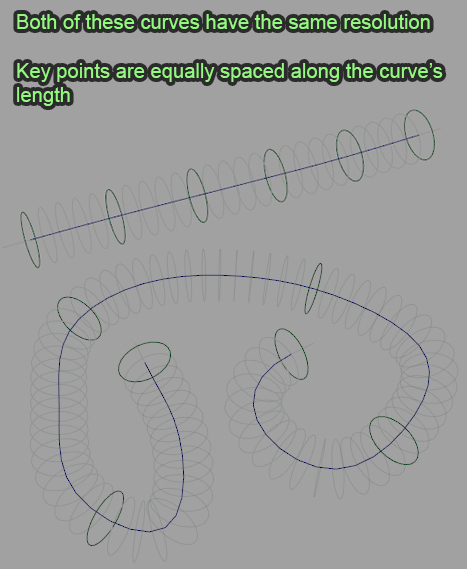## Editing a Curve Volume

There are 2 separate methods for editing a curve volume; editing its Radius Scale, and editing the key points.

The Radius Scale attribute affects the entire curve volume. It applies an overall scale to all of the key points, taking into account their relative radii. This attribute is handy to globally increase or decrease a curve volume very quickly.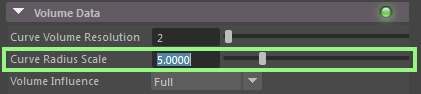### Editing Key Points

The key points define the radius at specific points along the curve. If you want a curve volume which is anything other than uniform, you will need to adjust the key points.

To edit a key point you will need to use the context manipulators which are provided on the VolumeLocatorNode. You can read more about this and how to edit key points in the Manipulators section.